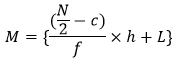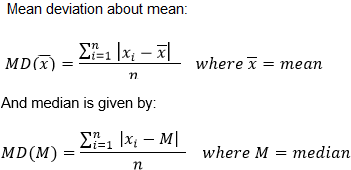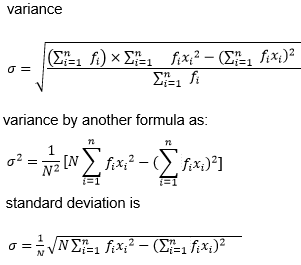# Statistics for IIT JEE

The word statistics is derived from the Latin word “status” which means a state. Statistics normally means taking the values of some parameters and by plotting it or by arranging it in a meaningful manner. Basically, statistics give us the process by which we can collect, analyze, interpret, present and organize data.

In statistics, we normally use words like “mean”, ”median” and “mode” to understand the data distributed throughout a range. Be it grouped or ungrouped, usually, these central tendency measures give a single data point which typically shows the behaviour of the whole data.

Also,

## Types of Averages

By averages, we normally mean the mean, median and mode of any data set.

### MEAN

Mean is the simple average of a data set ie mean can be represented by:

$\bar{x}$ = (sum of the observations)/(count of the observations)

In the mathematical sense, the mean is called average but all the times the two words don’t mean the same.

Let us take an example:

(In the case of discrete data)

The marks obtained by 10 students in a class are 56,54,89,74,23,16,94,52,68,100.

So, the mean of the data presented will be given by:

$\bar{x}$ = (56+54+89+74+23+16+94+52+68+100)/10 = 626/10 = 62.6

Now let us take the case of a grouped data.

The table shows the marks obtained by 10 students will be:

 Marks No. of students Mid-point(x) Value(x) 0-10 1 5 5 11-20 5 15.5 77.5 21-30 2 25.5 51 31-40 3 35.5 106.5 41-50 6 45.5 273 51-60 8 55.5 444 61-70 1 65.5 65.5 71-80 5 75.5 377.5 81-90 4 85.5 342 91-100 2 95.5 191

1st Step: We have to find the mid-points of every class and the interval is 10.

2nd Step: It will be the multiplication of the midpoint by the class frequency.

3rd Step: Then we sum the frequency and divide it by the frequency to get the mean.

$\bar{x}$ = (5+77.5+51+106.5+273+444+65.5+377.5+342+191)/10 = 1933/10 = 193.3

### MEDIAN

Median basically means the middle-most value in a data set. In case of an ungrouped data, firstly we will arrange the entire data set in ascending order. Now, if the number of terms is odd, we find the median by finding out the value of ((n+1)/2)th term and if the number of terms is even, the median will be given by the average of (n/2)th term and ((n+1)/2)th term

Median Calculation for grouped data:

Step 1: Find the class mark xi for each class.

Step 2: Find N = ∑ fi

Step 3: Take the median class to be that value whose cumulative frequency is near about (N/2)

Step 4: Calculate the median by the following value:L = lower limit of the median class

f = frequency of the median class

h = width of the median class

c = cumulative frequency of the class just preceding the median class

### MODE

By mode, we usually represent the maximum occurrence of a single element in a series of elements. This also represents a measure of central tendency. Sometimes, a series has only elements which have an occurrence of one time only, some have zero occurrences, and some have more than one.

## Dispersion in Statistics

By dispersion, we normally mean the extent to which the data is distributed or spread. It usually gives us a measure of the variation of every single data point from the average of all the points in a data set.

Now we can calculate dispersion by two types:

1. Absolute value of the dispersion
2. Relative value of the dispersion

### Absolute Value of Dispersion

This takes into account the expression of the data with respect to the original data. They cannot be used to compare two or more data sets and tell us whether the data is highly scattered or not.

 This includes: Range Mean Deviation Variance Standard Deviation

Range:

We normally find the range by subtracting the minimum value from the maximum value in a data set. We cannot tell how much the data are dispersed or scattered- so, to better that we introduce standard, mean and quartile deviation.

Mean Deviation:

The mean deviation tells us about the deviation from the mean or median data.

The formula of mean deviation is given by:Variance

Sometimes we have to take the mean deviation by taking the absolute values from a set of values. The absolute values were taken to measure the deviations, as otherwise, the positive and negative deviation may cancel out each other.

So, to remove the sign of deviation, we usually take the variance of the data set, i.e. we usually square the deviation values. As squares are always positive, so the variance is always a positive number.

Let us take “n” observations as a1, a2, a3, ….., an and their mean is represented by $\bar{a}$

Then the variance is denoted by

σ2 = $(a_1- \bar a)^2 +(a_2- \bar a)^2+(a_3- \bar a)^2 + …..+(a_n – \bar a)^2 = \sum_{i=1}^n (a_i- \bar{a})^2$

Properties of Variance

If the variance comes out to be zero, this means that (ai$\bar{a}$) is equal to zero, which is nothing but each value of the set is equal to the mean value $\bar{a}$.

If the variance is small, it means that the observations are pretty close to the mean value $\bar{a}$ and if the value is greater, the deviations of the observations are far from the mean value $\bar{a}$.

If each observation is increased by a where aϵR, then the variance will remain unchanged.

If each observation is multiplied by a where aϵR, then the variance will be multiplied by a2 also.

But for some data sets, the variance by the formula $\sum_{i=1}^n (a_i – \bar{a})^2$ does not give the proper values as the range of deviation may vary and the observations may be more scattered about the mean. So, to overcome this difficulty, we take the mean of the square of the deviations.

So, the variance is given by:

$\sigma^2 = \frac{1}{n} \sum_{i=1}^n (a_i – \bar a)^2$

As a result of squaring, the unit of variance is not the same as that of the data sets taken.

Standard Deviation

To take a proper measure of dispersion, we have to calculate the standard deviation by taking the square root of the variance. This measure often prevents above-average deviations from cancelling those below, which can sometimes contribute to a null variance. If the variance is great, then the standard deviation will be more, and for lesser variance, the opposite case occurs.

The formula of standard deviation is given by:

$\sigma = \sqrt{\sigma^2} = \sqrt{\frac{1}{n} \sum_{i=1}^n (a_i – \bar a)^2}$

Standard Deviation of distribution with discrete frequency:

It is given by:

$\sigma = \sqrt{\frac{1}{n} \sum_{i=1}^n (f_i a_i – \bar a)^2}$

Where the values are: a1, a2, a3 ,…., an

And the respective frequencies are: f1, f2, f3 ,…., fn

And N = $\sum_{i=1}^n f_i$

Standard Deviation of distribution with continuous frequency:## Relative Value of Dispersion

By this method, we represent the scattering in terms of some values ie either in percentage or in the form of a ratio. This is mainly helpful to compare three or more data sets all at a time.

 The different forms of the relative measures of dispersion are: ● Coefficient of Range ● Coefficient of Mean Deviation ● Coefficient of Variance and Standard Deviation

Coefficient of Range

It is defined as the ratio of the difference between the highest and lowest values to the sum of the two.

It is given by $\frac{x_m-x_o}{x_m+x_o }$

Coefficient of Mean Deviation

It is defined as the ratio of the mean deviation to the mean of the same data set.

It is given by (Mean deviation from Mean)/Mean or (Mean deviation from Median)/Median

## Solved Examples

Example 1: An experiment is conducted with 16 values of b, and the following results were obtained. ∑ b2 = 2560 and ∑ b = 180. On checking through the data again, it is seen that one observation with a particular value 30 is replaced with 20. What will be the corrected variance?

Solution:

Given: ∑ b2 = 2560 and ∑ b = 180

So, ∑ b1 = 180 – 30 + 20 = 170

And the variance will be decreased by ∑ b2 = 900 – 400 = 500

The value of variance becomes ∑ b2 = 2560 – 900 + 400 = 2060

So, the corrected variance = 1/n ∑ (b2 -[1/n ∑ b1]2 = 1/16 × 2060 – (1/16 × 170)2 = 128.75 – 112.890625 = 15.859375

Example 2: Calculate the median for the following data:

 Marks 0-10 10-20 20-30 30-40 40-50 No. of Students 5 15 20 2 8

Solution: First, we will calculate the cumulative frequency and mid-point.

 Marks Frequency Cumulative frequency Mid-point 0-10 5 5 5 10-20 15 20 15 20-30 20 40 25 30-40 2 42 35 40-50 8 50 45

Thus, N = 50 and N/2 = 25

So, the median class is 20-30.

L = 20, f = 20, h = 10 and c = 20

So, median = (25-20)/20 × 10 + 20 = 22.5

Example 3:

Let us take two sets of values where one set is represented by the scores of 100 Indian batsmen, and the other represents the scores of 100 Australian batsmen. Incidentally, the Indians have scored runs in the order 550, 551, 552, ….., 649. And the Australian batsmen have scored runs in the order 900, 901, 902, …., 999. If the variances of the two sets are represented by σA and σB, then what will be the value of σAB.

Solution:

We know, $\sigma^2 = \frac{\sum d_i^2}{n}$

Here, both the Australian and Indian Batsmen set have 100 consecutive positive integers and the value of n = 100, which is also the same. Thus, $\sum d_i^2$ is the same for both of these integer sets.

So, σAB =1

Example 4: The S.D. of a variate x is s. The S.D. of the variate $\frac{ax+b}{c}$ where a, b, c are constant, is

$A) \left( \frac{a}{c} \right)\,\sigma\\ B) \left| \frac{a}{c} \right|\,\sigma\\ C) \left( \frac{{{a}^{2}}}{{{c}^{2}}} \right)\,\sigma \\ D) \text \ None \ of \ these$

Solution:

Let

$y=\frac{ax+b}{c}\\ y=\frac{a}{c}x+\frac{b}{c}\\ y=Ax+B,$

where

$A=\frac{a}{c},B=\frac{b}{c} \\ \bar{y}=A\bar{x}+B\\ y-\bar{y}=A(x-\bar{x})\\ {{(y-\bar{y})}^{2}}={{A}^{2}}{{(x-\bar{x})}^{2}}\\ \sum {{(y-\bar{y})}^{2}}={{A}^{2}}\sum {{(x-\bar{x})}^{2}}\\ n.\sigma _{y}^{2}={{A}^{2}}.n\sigma _{x}^{2}\\ \sigma _{y}^{2}={{A}^{2}}\sigma _{x}^{2}\\ {{\sigma }_{y}}=\,|A|{{\sigma }_{x}}\\ {{\sigma }_{y}}=\,\left| \frac{a}{c} \right|{{\sigma }_{x}}$

Example 5: Let r be the range and ${{S}^{2}}=\frac{1}{n-1}\sum\limits_{i=1}^{n}{{{({{x}_{i}}-\bar{x})}^{2}}}$ be the S.D. of a set of observations ${{x}_{1}},\,{{x}_{2}},\,…..{{x}_{n}},$ then

$A) S\le r\sqrt{\frac{n}{n-1}}\\ B) S=r\sqrt{\frac{n}{n-1}}\\ C) S\ge r\sqrt{\frac{n}{n-1}}\\ D) \text \ None \ of \ these$

Solution:

We have

$\underset{\,\,\,\,\,\,\,\,\,\,\,\,\,i\,\ne j}{\mathop{r=\max |{{x}_{i}}-{{x}_{j}}|}}\,$ and ${{S}^{2}}=\frac{1}{n-1}\sum\limits_{i=1}^{n}{{{({{x}_{i}}-\bar{x})}^{2}}}$

Now

${{({{x}_{i}}-\bar{x})}^{2}}={{\left( {{x}_{i}}-\frac{{{x}_{1}}+{{x}_{2}}+…..+{{x}_{n}}}{n} \right)}^{2}}\\ =\frac{1}{{{n}^{2}}}[({{x}_{i}}-{{x}_{1}})+({{x}_{i}}-{{x}_{2}})+….+({{x}_{i}}-{{x}_{i}}-1)\\ +({{x}_{i}}-{{x}_{i}}+1)+…….+({{x}_{i}}-{{x}_{n}})]\le \frac{1}{{{n}^{2}}}{{[(n-1)r]}^{2}},[\text \ because |{{x}_{i}}-{{x}_{j}}|\le r]\\ {{({{x}_{i}}-\bar{x})}^{2}}\le {{r}^{2}}\Rightarrow \sum\limits_{i=1}^{n}{{{({{x}_{i}}-\bar{x})}^{2}}\le n{{r}^{2}}}\\ \frac{1}{n-1}\sum\limits_{i=1}^{n}{{{({{x}_{i}}-\bar{x})}^{2}}\le \frac{n{{r}^{2}}}{(n-1)}}\\ {{S}^{2}}\le \frac{n{{r}^{2}}}{(n-1)}\\ S\le r\sqrt{\frac{n}{n-1}}.$

Example 6: The mean and S.D. of the marks of 200 candidates were found to be 40 and 15 respectively. Later, it was discovered that a score of 40 was wrongly read as 50. The correct mean and S.D. respectively are

A) 14.98, 39.95

B) 39.95, 14.98

C) 39.95, 224.5

D) None of these

Solution:

Corrected $\Sigma x=40\times 200-50+40=7990$

Corrected $\bar{x}=7990/200=39.95$

Incorrect $\Sigma {{x}^{2}}=n\,[{{\sigma }^{2}}+{{\bar{x}}^{2}}]=200[{{15}^{2}}+{{40}^{2}}]=365000$

Correct $\Sigma {{x}^{2}}=365000-2500+1600=364100$

Corrected $\sigma = \sqrt{\frac{364100}{200}-{{(39.95)}^{2}}}\\=\sqrt{(1820.5-1596)}\\=\sqrt{224.5}\\=14.98.$

Example 7: The average of n numbers ${{x}_{1}},\,{{x}_{2}},\,{{x}_{3}},\,……,\,{{x}_{n}}$ is M. If ${{x}_{n}}$ is replaced by ${x}'$, then new average is

$A) M-{{x}_{n}}+{x}’\\ B) \frac{nM-{{x}_{n}}+{x}’}{n}\\ C) \frac{(n-1)M+{x}’}{n}\\ D) \frac{M-{{x}_{n}}+{x}’}{n}$

Solution:

$M=\frac{{{x}_{1}}+{{x}_{2}}+{{x}_{3}}……{{x}_{n}}}{n}\\ \underset{n}{\mathop{\underline{\begin{matrix} nM \\ nM-{{x}_{n}} \\ nM-{{x}_{n}}+{x}’ \\ \end{matrix}}}}\,\begin{matrix} = \\ = \\ = \\ \end{matrix}\underset{n}{\mathop{\underline{\begin{matrix} {{x}_{1}}+{{x}_{2}}+{{x}_{3}}+……{{x}_{n-1}}+{{x}_{n}} \\ {{x}_{1}}+{{x}_{2}}+{{x}_{3}}+……{{x}_{n-1}}\ \ \ \ \ \\ {{x}_{1}}+{{x}_{2}}+{{x}_{3}}+……{{x}_{n-1}}+{x}’ \\ \end{matrix}}}}\,$

New average $=\frac{nM-{{x}_{n}}+{x}’}{n}$